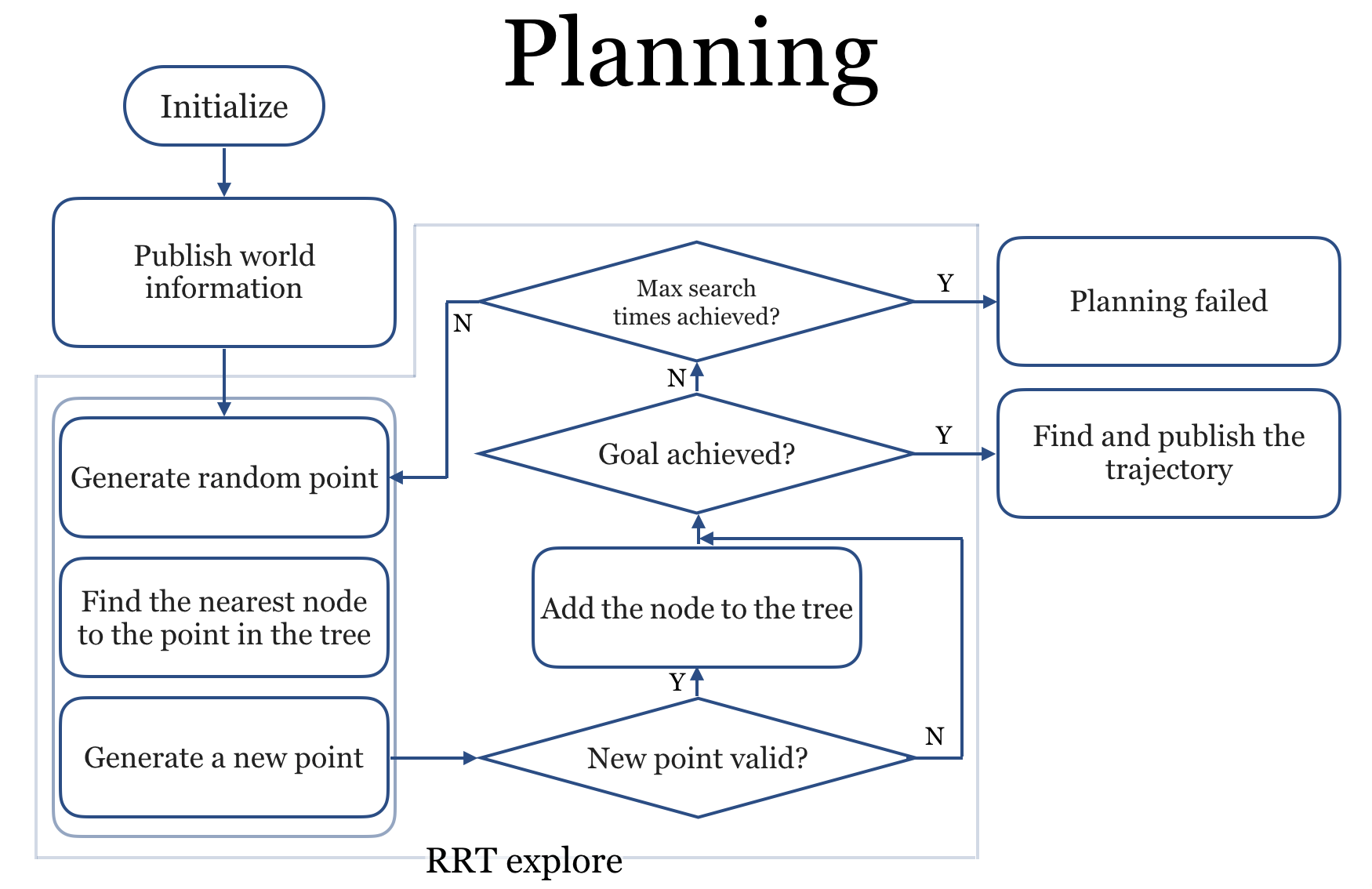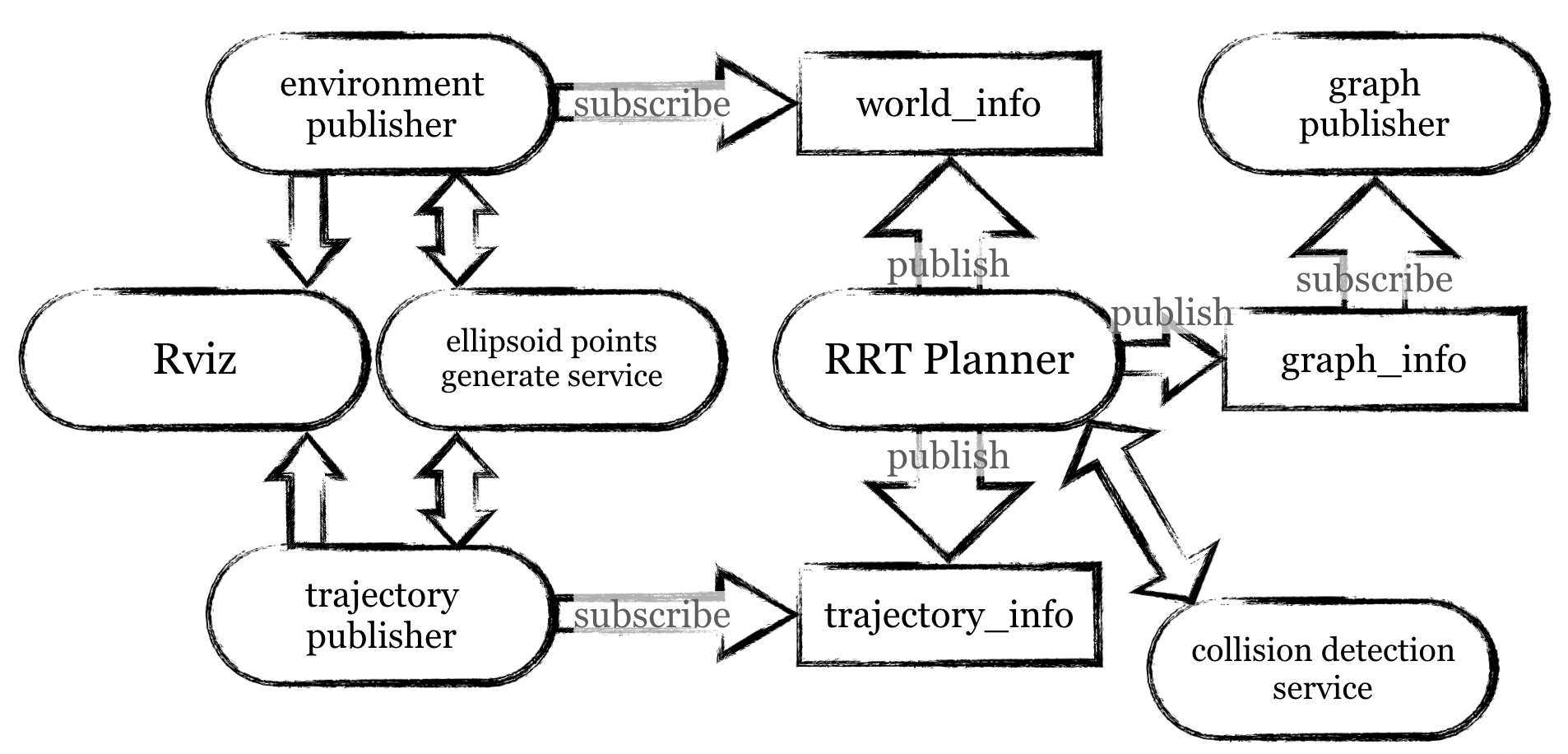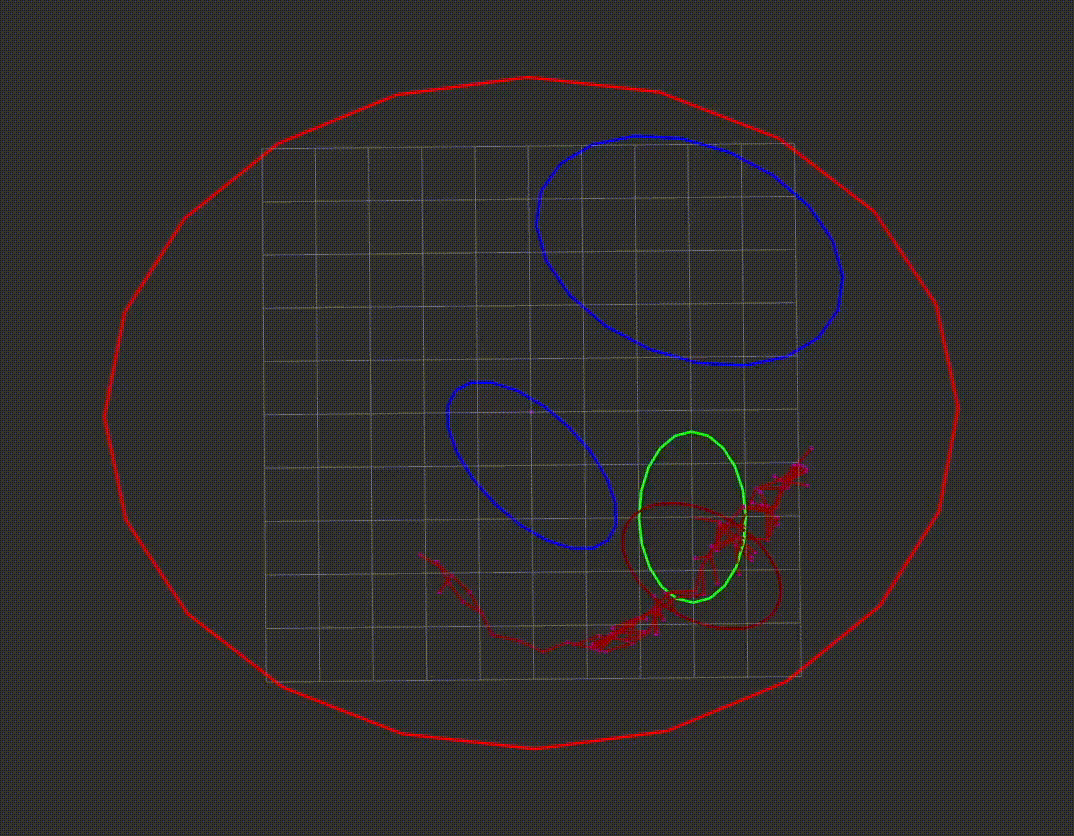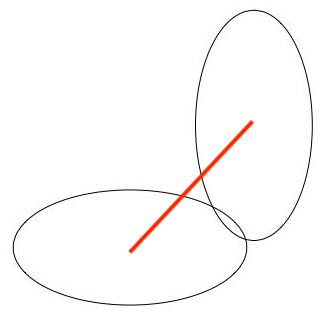# Implementation of RRT Motion Planning

• This is a project I completed in the summer of 2018, under Karen and Sipu’s help, in Johns Hopkins University.
• This can be found on my github page.
• This is an implementation of Rapid-exploring Random Tree Algorithm on ROS, in 2D plane and the robot, obstacles and world are all in a shape of ellipsoid. The main language is C++.

## Motion Planning with RRT

The procedure of RRT planner is described below:

• Initialize: build a RRT tree whose root is the start point (in a n-dimension space) of the motion. Every node in the tree represents a “valid” point in the C-Space, which means under that position the robot is not in collision.

• Explore the C-Space and grow the tree: (loop)

• Generate a random pose of the ellipsoid robot ($q_{rand}$)
• Traverse all the node in the tree and find a $q_{nearest}$ , which is nearest to the $q_{rand}$
• Generate a new point $q_{new}$ which is between $q_{nearest}$ and $q_{rand}$ and is a metric away from $q_{nearest}$
• IF ( $q_{new}$ is valid ) THEN { add $q_{new}$ to the tree}. Once the goal is achieved or the search time is more than the set upper limit, the search should be terminated.• Find the trajectory from the generated tree, if the goal is successfully achieved. Dijkstra Algorithm can be used.

• Smooth the trajectory.## The Structure of My ROS Package

#### msg

• ellipsoid.msg: ellipsoid semi-axes length, central point ordinates value, angle
• world.msg: describe the arena, obstacles and robot with ellipsoid message
• position.msg: (x, y, 𝜙) value describing the ellipsoid pose
• point.msg: (x, y) value describing the 2D point
• trajectory.msg: an array of (x, y) describing the points on the trajectory
• pointsArrary.msg: an array of (x, y) on the boundary of ellipsoid generated by the server
• node.msg: the x, y values of the valid searched node, father value represents the nearest node ID
• rrtGraph.msg: array of node

#### srv

• collision_detect.srv: return whether the state is in collision according to the world msg information and current robot position msg
• ellipsoid_points.srv: return a vector of point msg according to the ellipsoid msg and step

#### include/rrt_implement

• rrt.h: define the basic tree structure
• planner.h: define the planning action

#### src

• rrt.cpp
• planner.cpp: plan the motion. I use Euler distance to find the nearest node —— rrtPlanner
• collision_detect.cpp: provide the service of collision detection —— collision_detection_srv
• environment_publisher.cpp: subscribe the world information published by node rrtPlanner, and then send that to the server ellipsoid_point_gen_srv, publish the obtained 2D points to be plotted —— environment_pub
• graph_publisher.cpp: subscribe the graph information published by node rrtPlanner, and then publish—— graph_pub
• trajectory_publisher.cpp: subscribe the trajectory information published by node rrtPlanner, and then send that to the server ellipsoid_point_gen_srv, publish the obtained 2D points to be plotted —— trajectory_pub
• ellipsoid_point_gen.cpp: a server whose input is ellipsoid msg and position msg and output is pointsArray msg describing the boundary of the ellipsoid —— ellipsoid_point_gen_srv## Run the Demo

#### RVIZ configuratoin

##### Global Optional
• Fixed Frame = “/root”
• arena
• obstacle0
• obstacle1
• robot
• trajectory
• graph

#### Commands

after catkin_make && source devel/setup.bash

• run the server
• run the rrt planner
• run the publisher
• run rviz

#### Running Result## Collision Detection

To achieve high speed, I did not call the collision detection libraries such as FCL. I just used a simple and rough method. Assume that $A$, $B$ is the center of two ellipsoids, respectively, and their distance is D. The “radius”s of the two ellipsoids in the direction of $\overrightarrow{AB}$ is $R$ and $r$, respectively. Thus, the requirement of the two ellipsoids is seperate is $D>R+r$; the requirement of the ellipsoid $B$ is in ellipsoid $A$ entirely is $D<R-r$.

As this is just the requirement, sometimes this method would fail.Share